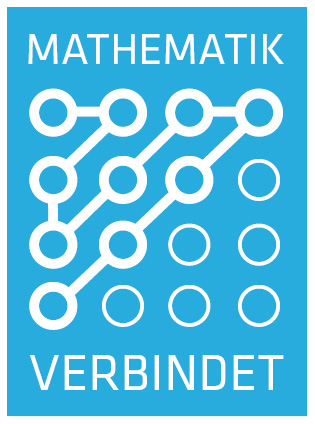# Recent Publications

### Application of Randomized Quadrature Formulas to the Finite Element Method for Elliptic Equations

The implementation of the finite element method for linear elliptic equations requires to assemble the stiffness matrix and the load …

### A randomized Milstein method for stochastic differential equations with non-differentiable drift coefficients

In this paper a drift-randomized Milstein method is introduced for the numerical solution of non-autonomous stochastic differential …

### Error Estimates of the Backward Euler-Maruyama Method for Multi-Valued Stochastic Differential Equations

In this paper, we derive error estimates of the backward Euler-Maruyama method applied to multi-valued stochastic differential …

### Two quadrature rules for stochastic Itô-integrals with fractional Sobolev regularity

In this paper we study the numerical quadrature of a stochastic integral, where the temporal regularity of the integrand is measured in …

### A randomized and fully discrete Galerkin finite element method for semilinear stochastic evolution equations

In this paper the numerical solution of nonautonomous semilinear stochastic evolution equations driven by an additive Wiener noise is …

# How to find us

• Georg-Cantor-Haus
Theodor-Lieser-Str. 5
06120 Halle (Saale)
• Enter the Georg-Cantor-Haus and take the stairs to the first floor. Our offices are located at the end of the long corridor.